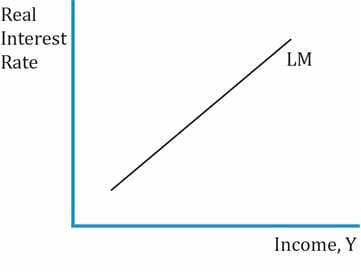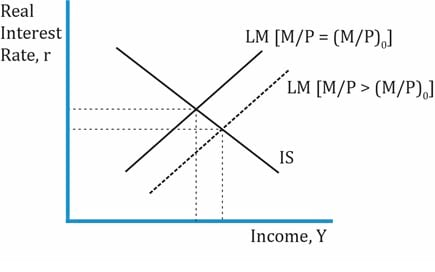IFT Notes for Level I CFA® Program
IFT Notes for Level I CFA® Program

# Part 4

Equilibrium in the Money Market: the LM Curve

Key points related to the LM curve as follows:

• The price level depends on the supply and demand of money in the economy.
• Money supply is exogenous. It is determined by the central bank.
• Equilibrium in the money market means the demand for money equals the supply of money.
• Quantity theory of money is a theory of money supply and demand. It equates nominal money supply, the price level, and real income. It is expressed as:

MV = PY

It can be rewritten as:where

M = nominal money supply

P = price level (or GDP deflator)

Y = real income/expenditure

V = velocity of money = average rate at which money circulates through the economy to facilitate expenditure. In simple words, it is the number of times money supply is used to purchase goods and services.

Quantity theory of money makes sense with the following assumptions:

• V is constant. If velocity is constant, money supply determines the nominal value of output (P * Y) (nominal GDP). Increase in money supply increases price level and nominal value of output.
• Output (Y) is independent of M. Recall from the Solow growth model that Y is a function of A, L, and K.
• Price level is determined by M and not the other way round, because M is an exogenous variable and money supply is determined by the central bank. Therefore, any change in M is directly proportional to change in P.

Relationship between money and interest rates:

• Demand for money is inversely related to interest rates because:
• The other avenues for investment other than bank deposits are stocks and bonds. Higher interest rates will mean lower demand for real money. Investors would shift their money from bank deposits to stock and bonds. If interest rates are low, then demand for money will be high.
• People hold money in relation to their income, and the opportunity cost of holding money. So, higher income means higher money balances.

Summary: LM CurveDemand for real money balances is positively related to real income.

Demand for real money is inversely related to the interest rate.

If real money supplyis constant, then there is a positive relationship between interest rates and income.Interpretation of the graph:

• The LM curve is based on the premise that there is equilibrium in the money market.
• It plots real income and output on the x-axis and real interest rate on the y-axis.
• Shows positive relationship between interest rates and income: upward sloping curve.

The IS and LM Curves:

The graph below shows the intersection of the IS and LM curve.• The intersection of IS and LM curves shows the real income and real interest rate that satisfies two equilibrium conditions: aggregate income = planned expenditure (IS curve) and money supply = demand for money (LM curve).
• The dashed line in the graph shows higher supply of real money (decrease in the price level).
• Decrease in price level à increase in the supply of real money à there is a shift in the LM curve to the right. When the price goes down, real income is higher because goods and services are cheaper. The equilibrium point occurs at a higher level of real income and lower level of real interest rate.

How the IS and LM Curves can be used to generate the AD curve

The graph on the left shows the IS and LM curves. It plots income and output against real interest rate.

The graph to the right shows the aggregated demand curve. It plots income, output, and Y against price level.

• IS-LM curve:
• Assume the initial price level to be 100 and the interest rate as 5%. The income at this point is 200.
• Now, if the price level comes down to 90, the LM curve shifts to the right. The income is higher now at 210 and the new interest rate is 4%. The equilibrium point moves from A to B.
• If you plot the (price level, income) points of (100,200) and (90,210), you will notice that the AD curve is downward sloping.

For a movement along the AD curve, these two equilibrium conditions must hold good:

• (S – I) = (G – T) + (X – M); assuming no changes in trade and fiscal balance, changes in savings = changes in investment spending.
• Equilibrium in the money market: money supply = money demand.

Effect on increase in price level:

• If price level increases, real money supply decreases. Recall money supply =.
• If equilibrium condition real money supply = real money demand must hold good, then real money demand must decrease. For demand to decrease, interest rates must increase. When interest rates rise, demand for other investments such as stocks/bonds goes up and demand for money decreases.
• Income and demand for money have a direct relationship. So for demand to decrease, income must fall, and so would the need for real money balances.
• Increase in interest rates will reduce investment spending, because it’s expensive for businesses to borrow money.

Note: Here are a few practice questions. The concepts have been repeated as part of the solution – just for reinforcement. The more you do these types of questions, the clearer you will be with these concepts.

Example

1. Which curve represents the combinations of income and real interest rates at which planned expenditure = income?
2. Solution: IS curve
1. What is the relationship between investments and real interest rates?
2. Solution: Inversely related. Negative relationship
1. What is the relationship between investments and aggregate output?
2. Solution: Positive relationship.
1. Which curve represents the combinations of income and real interest rates such that the supply of real money = demand for real money?
2. Solution: LM curve
1. If there is an increase in government spending, what is the impact on IS, LM and AD curves?
2. Solution: IS curve: derived by plotting the intersection of upward sloping S-I curve and downward sloping (G – T) + (X – M) curves. This graph has a real interest rate (y-axis) vs. real income/expenditure (x-axis). If government spending goes up, then (G – T) goes up. The (G – T) + (X – M) curve shifts to the right.
1. LM curve: not impacted by government spending.
2. AD curve: derived by plotting the intersection of IS and LM curves. Plots price level on the y-axis and output on the x-axis. AD curve will also shift to the right because IS shifts to the right.
1. If there is an increase in nominal money supply, what is the impact on the IS, LM, and AD curves?
2. Solution: An increase in nominal money supply means there is an increase in real money supply as well. This shifts the LM curve to the right. Increase in real money supply → lower real interest rates → higher real income → expenditure has increased.
3. There is no impact on the IS curve.
4. At a given price level, since the expenditure has increased, the aggregate demand curve also shifts to the right.
1. An increase in the price level would shift which of the following curves: IS, LM, AD? In what direction?
2. Solution: Increase in price level → real money supply decreases.
3. The LM curve shifts to the left. The new equilibrium point to derive the AD would be at a lower income level and a higher interest rate.
4. Since the AD is derived from the intersection of IS and LM curves, there would not be any shift. It will just be a movement along the AD curve. There is no impact to the IS curve.
1. If there is a movement along the AD curve with output increasing, what is the likely direction of interest rates?
2. Solution: Output is increasing → price level is coming down.
3. If the price level decreases, then LM curve shifts to the right.
4. For a given IS curve, when the LM curve shifts to the right, the interest rate comes down.

Note: The learning objectives do not explicitly mention the slope of the AD curve. However, the curriculum covers this material at the end of section 3.1. To be safe, the main points are being reproduced here:

The slope of the AD curve is determined by the relative sensitivities of investment, saving and money demand to income and the interest rate. The AD curve is flatter if:

• Investment expenditure is highly sensitive to the interest rate.
• Saving is insensitive to income.
• Money demand is insensitive to interest rates.
• Money demand is insensitive to income.